# Precalculus : Trigonometric Functions

## Example Questions

### Example Question #4 : Convert Between Degrees And Radians

Convertto radians.

Possible Answers: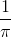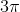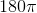Correct answer:Explanation:

To solve this problem, we need to know that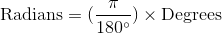we can arrive to our answer by using this formula: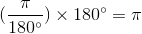### Example Question #5 : Convert Between Degrees And Radians

Convert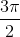to degrees.

Possible Answers: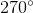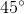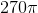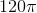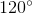Correct answer:Explanation:

In order to solve this problem, we need to know that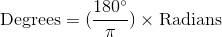we can arrive to our answer by using this formula: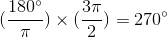### Example Question #6 : Convert Between Degrees And Radians

Findexpressed as radians.

Possible Answers: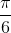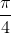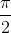Correct answer:Explanation:

To convert from degrees into radians,

multiply the angle in degrees by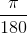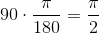### Example Question #7 : Convert Between Degrees And Radians

Convert the following to degrees: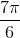Possible Answers: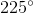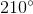Correct answer:Explanation:

To convert, multiply by the conversion factor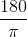.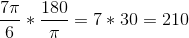### Example Question #8 : Convert Between Degrees And Radians

Convert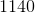degrees into radians.

Possible Answers: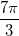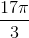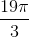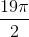Correct answer:Explanation:

To convert between degrees and radians, you must use the following conversion factor 180 degrees = pi radians.

Therefore: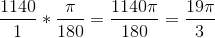### Example Question #1 : Convert Between Degrees And Radians

Please convert the following from degrees to radians: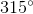Possible Answers: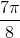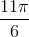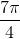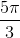Correct answer:Explanation:

To convert from degrees to radians, multiply the input by:In this case: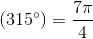### Example Question #10 : Convert Between Degrees And Radians

Please convert the following angle to degrees:Possible Answers: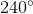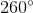Correct answer:Explanation:

To convert from radians to degrees, multiply the input by.

In this case: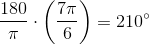### Example Question #11 : Angle Measures In Degrees And Radians

Convertradians to degrees.  Round to the nearest integer.

Possible Answers: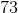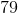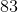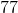Correct answer:Explanation:

In order to convert from radians to degrees, we will need to know how many degrees are in a radian.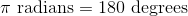Substitute 180 as a replacement for.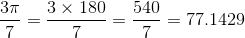The correct answer is.

### Example Question #12 : Angle Measures In Degrees And Radians

Convert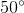to radians.

Possible Answers: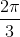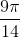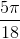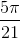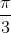Correct answer:Explanation:

To convert degrees to radians we must recall the conversion factor. Remember that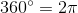.

Therefore, we can create the following fraction and solve for the missing variable.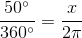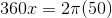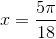### Example Question #13 : Angle Measures In Degrees And Radians

Megan, a civil engineer, measures the angle made by the angle of a road. She finds the angle to be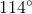. What is the angle in radians?

Possible Answers: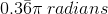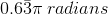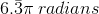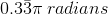Correct answer:Explanation:

Megan, a civil engineer, measures the angle made by the angle of a road. She finds the angle to be. What is the angle in radians?

To convert from degrees to radians, use the following formula: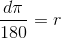Whereandstand for degrees and radians, respectively.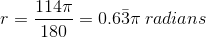So we get### All Precalculus Resources